# Hierarchical Clustering in R Programming

• Last Updated : 03 Dec, 2021

Hierarchical clustering in R Programming Language is an Unsupervised non-linear algorithm in which clusters are created such that they have a hierarchy(or a pre-determined ordering). For example, consider a family of up to three generations. A grandfather and mother have their children that become father and mother of their children. So, they all are grouped together to the same family i.e they form a hierarchy.

## R – Hierarchical Clustering

Hierarchical clustering is of two types:

Attention reader! Don’t stop learning now. Get hold of all the important Machine Learning Concepts with the Machine Learning Foundation Course at a student-friendly price and become industry ready.

• Agglomerative Hierarchical clustering: It starts at individual leaves and successfully merges clusters together. Its a Bottom-up approach.
• Divisive Hierarchical clustering: It starts at the root and recursively split the clusters. It’s a top-down approach.

### Theory:

In hierarchical clustering, Objects are categorized into a hierarchy similar to a tree-shaped structure which is used to interpret hierarchical clustering models. The algorithm is as follows:

1. Make each data point in a single point cluster that forms N clusters.
2. Take the two closest data points and make them one cluster that forms N-1 clusters.
3. Take the two closest clusters and make them one cluster that forms N-2 clusters.
4. Repeat steps 3 until there is only one cluster.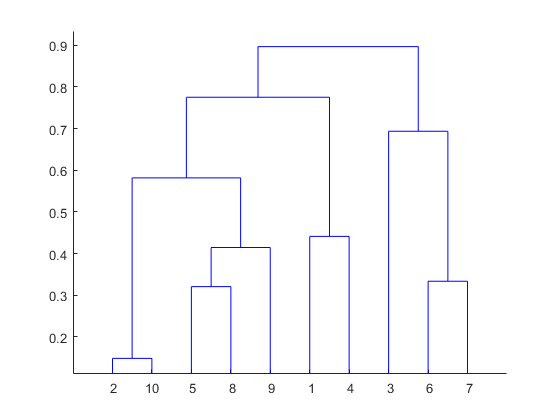Dendrogram is a hierarchy of clusters in which distances are converted into heights. It clusters n units or objects each with p feature into smaller groups. Units in the same cluster are joined by a horizontal line. The leaves at the bottom represent individual units. It provides a visual representation of clusters.
Thumb Rule: Largest vertical distance which doesn’t cut any horizontal line defines the optimal number of clusters.

## The Dataset

mtcars(motor trend car road test) comprise fuel consumption, performance, and 10 aspects of automobile design for 32 automobiles. It comes pre-installed with dplyr package in R.

## R

 `# Installing the package``install.packages``(``"dplyr"``)``  ` `# Loading package``library``(dplyr)``  ` `# Summary of dataset in package``head``(mtcars)`

Output: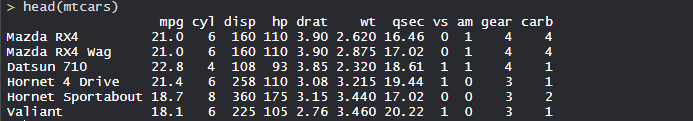## Performing Hierarchical clustering on Dataset

Using Hierarchical Clustering algorithm on the dataset using hclust() which is pre-installed in stats package when R is installed.

## R

 `# Finding distance matrix``distance_mat <- ``dist``(mtcars, method = ``'euclidean'``)``distance_mat` `# Fitting Hierarchical clustering Model``# to training dataset``set.seed``(240)  ``# Setting seed``Hierar_cl <- ``hclust``(distance_mat, method = ``"average"``)``Hierar_cl` `# Plotting dendrogram``plot``(Hierar_cl)` `# Choosing no. of clusters``# Cutting tree by height``abline``(h = 110, col = ``"green"``)` `# Cutting tree by no. of clusters``fit <- ``cutree``(Hierar_cl, k = 3 )``fit` `table``(fit)``rect.hclust``(Hierar_cl, k = 3, border = ``"green"``)`

Output:

• Distance matrix: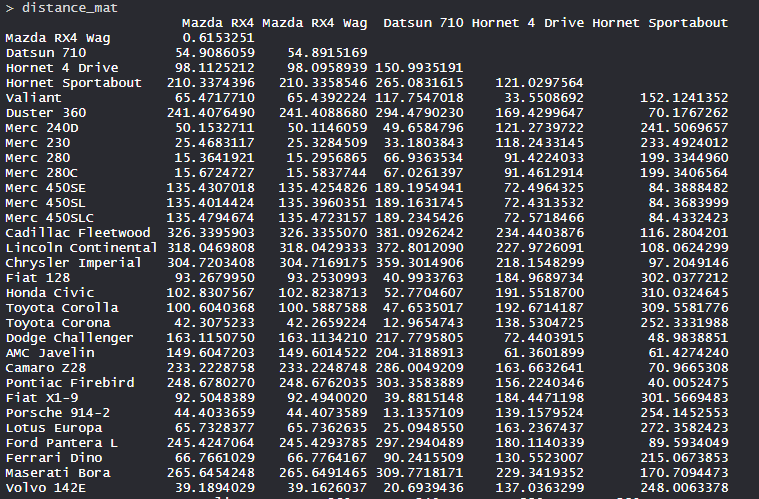• The values are shown as per the distance matrix calculation with the method as euclidean.
• Model Hierar_cl: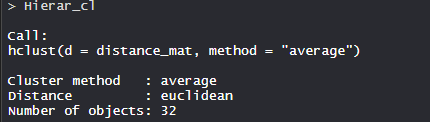• In the model, the cluster method is average, distance is euclidean and no. of objects are 32.
• Plot dendrogram: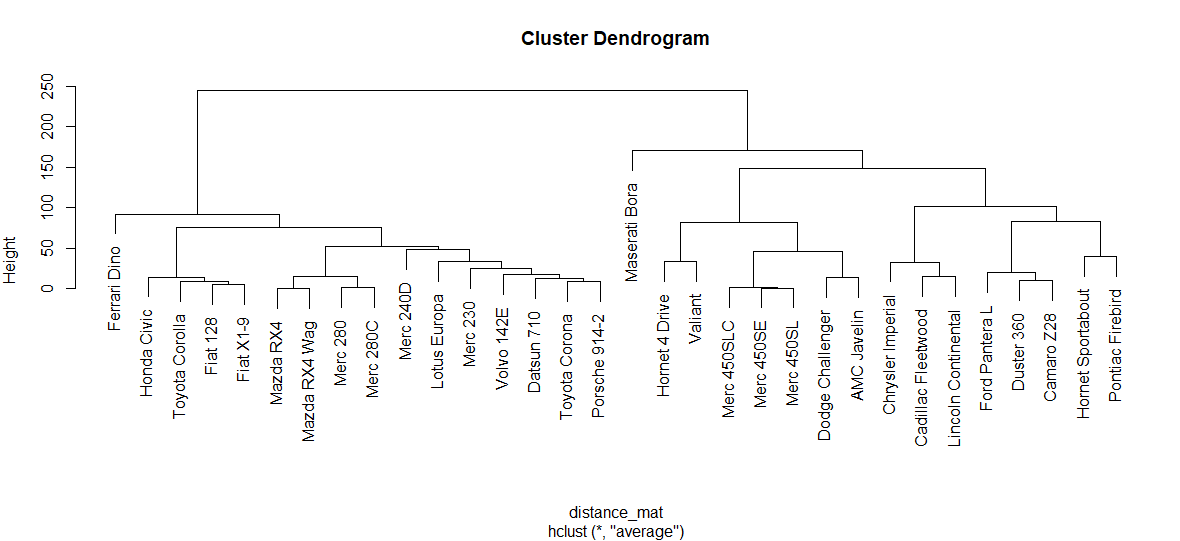• The plot dendrogram is shown with x-axis as distance matrix and y-axis as height.
• Cutted tree: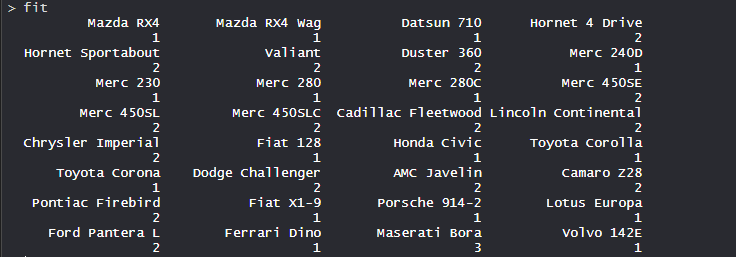• So, Tree is cut where k = 3 and each category represents its number of clusters.
• Plotting dendrogram after cutting: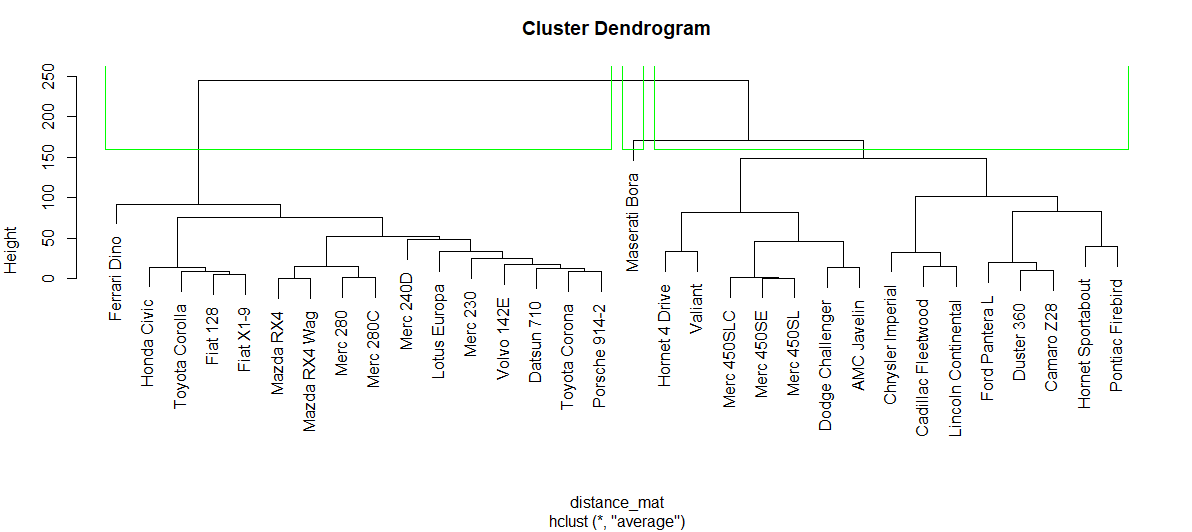• The plot denotes dendrogram after being cut. The green lines show the number of clusters as per the thumb rule.

My Personal Notes arrow_drop_up